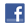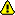Get 1 free lesson per week // Add a new lessonNew account
4 million accounts created!

• Home
• Print
• Guestbook
• Report a bug

•Get a free English lesson every week!

Partners:
- Our other sites

Learn English > English lessons and exercises > English test #47116: Numbers and calculation> Other English exercises on the same topic: Numbers [Change theme] > Similar tests: - Cardinal and ordinal numbers - Fractions and percentages - ESL-Numbers from 1 to 10 - Geometric shapes - Vocabulary: mathematics - Geometry-Vocabulary - Idioms: numbers - Numbers and dates... (Level 1) > Double-click on words you don't understand

# Numbers and calculation

CALCULATION

 10                +               5                   =                  15Ten            plus            five               equals           fifteen Ten plus five equals fifteen. 22                        -                     11                   =                  11Twenty-two      minus              eleven             equals            eleven Twenty-two minus eleven equals eleven. 17                       x                         3                     =                 51Seventeen       multiplied by          three              equals            fifty-one Seventeen multiplied by three equals fifty-one. 15                     ÷                          5                     =                  3Fifteen            divided by           five                  equals           three Fifteen divided by five equals three.Twitter Share
English exercise "Numbers and calculation" created by anonyme with The test builder.Please log in to save your progress.

1. Twenty-three seven equals thirty.

2. multiplied by ten equals sixty.

3. Fifty-six two equals one hundred and twelve.

4. Three thousand two thousand equals one thousand.

5. Eighty-eight plus two equals .

6. Six three equals two.

7. Four hundred four hundred equals one.

8. Seven minus equals six.

9. Ninety-nine five equals one hundred and four.

10. One million ten thousand equals one hundred.

End of the free exercise to learn English: Numbers and calculation
A free English exercise to learn English.
Other English exercises on the same topic : Numbers | All our lessons and exercises